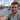# Command line arguments parser

June 17, 2009

There are already 2 or 3 command line arguments in C#, two of which are found on the codeproject.com website. Both of these didn’t match my exact needs so I decided to write my own one.

An updated and more advanced parser can be found in the CommandOptions class.

The format is the Unix bash command line format, not the Windows forward slash format.

The one below doesn’t use regular expressions (mostly from realising I could do it faster tokenizing than failing with the regex syntax for hours). It’s implemented using a basic state machine, it could probably be improved to use the State pattern, but works fine for now. I haven’t researched the optimum way for token parsing, as I’m sure there are proven methods for doing it. If you know any sites or examples of these (Douglas Crokford’s JSLint is one example I know), contact me on the contact page.

Without specifying the args, the class automatically grabs the command line from the System.Environment.CommandLine property, and removes the current process name from this.

Correct formats it accepts are:

-arg=value
-arg1=value -arg2=value
-arg=‘my value’
-arg=”my value”
-arg=1,2,3,4 -arg2=another

Incorrect formats it won’t accept are:

-arg1 = value
—arg1=value
-arg1 value
-arg1 “value value”

Here’s the source, with example usage:

This file contains bidirectional Unicode text that may be interpreted or compiled differently than what appears below. To review, open the file in an editor that reveals hidden Unicode characters. Learn more about bidirectional Unicode characters
 static void Main(string[] args) { string[] example = { @"--arg1=""test"" --arg2= this is wrong --arg3=x --arg4=""value 4""" }; // Default usage CommandLineTokenizer tokenizer = new CommandLineTokenizer(example); Console.WriteLine(tokenizer["arg1"]); Console.WriteLine(tokenizer["arg2"]); // bad tokens are blank strings Console.WriteLine(tokenizer["arg5"]); // unknown tokens will also be blank string // Alternative usage tokenizer = new CommandLineTokenizer(); List> list = tokenizer.Tokenize(example); } public class CommandLineTokenizer { private enum State { None, FirstDash, SecondDash, StartArg, StartArgDoubleQuotes, EndArgDoubleQuotes, StartArgSingleQuotes, EndArgSingleQuotes, Parse } private List> _tokens; public string this[string key] { get { for (int i = 0; i < _tokens.Count; i++) { if (_tokens[i].Key == key) return _tokens[i].Value; } return ""; } } public CommandLineTokenizer() { string line = Environment.CommandLine; line = line.Replace("\"" + Process.GetCurrentProcess().MainModule.FileName + "\"", ""); _tokens = Tokenize(line); } public CommandLineTokenizer(string[] args) : this() { string input = string.Join(" ", args); _tokens = Tokenize(input); } public List> Tokenize(string input) { return Pass2(Pass1(input)); } private List Pass1(string input) { State state = State.None; int start = 0; int end = 0; List list = new List(); for (int i = 0; i < input.Length; i++) { char current = input[i]; //Console.WriteLine("{0} {1}", current, state); if (input[i] == '-') { if (state == State.None) { state = State.FirstDash; } else if (state == State.FirstDash) { state = State.SecondDash; } else if (state == State.SecondDash) { state = State.None; start = 0; } } else if (state == State.SecondDash) { start = i; state = State.StartArg; } else if (current == '"') { if (state == State.StartArg) { state = State.StartArgDoubleQuotes; } else if (state == State.StartArgDoubleQuotes) { state = State.EndArgDoubleQuotes; if (i == input.Length - 1) { state = State.Parse; end = input.Length; } } } else if (current == '\'') { if (state == State.StartArg) { state = State.StartArgSingleQuotes; } else if (state == State.StartArgSingleQuotes) { state = State.EndArgSingleQuotes; if (i == input.Length - 1) { state = State.Parse; end = input.Length; } } } else if ((state == State.EndArgSingleQuotes || state == State.EndArgDoubleQuotes) && (current == ' ' || i == input.Length - 1)) { end = i + 1; state = State.Parse; } else if (state == State.StartArg && (current == ' ' || i == input.Length - 1)) { end = i + 1; // get past this current char state = State.Parse; } if (state == State.Parse) { state = State.None; list.Add(input.Substring(start, end - start)); } } return list; } private List> Pass2(List list) { List> result = new List>(); foreach (string item in list) { int equals = item.IndexOf("="); if (equals > -1 && item.Length >= 3) { string key = item.Substring(0, equals); string value = item.Substring(equals + 1); KeyValuePair pair = new KeyValuePair(key, value); result.Add(pair); } } return result; } }I'm Chris Small, a software engineer working in London. This is my tech blog. Find out more about me via GithubStackoverflowResume